### 15.2.1.2 The continuous case

Now consider adapting to the continuous case. Supposeand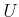are both continuous, but discrete stages remain, and verify that (15.5) to (15.8) still hold true. Their present form can be used for any system that is approximated by discrete stages. Suppose that the discrete-time model of Section 14.2.2 is used to approximate a system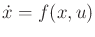on a state spacethat is a smooth manifold. In that model,was discretized to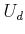, but here it will be left in its original form. Let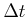represent the time discretization.

The HJB equation will be obtained by approximating (15.6) with the discrete-time model and lettingapproach zero. The arguments here are very informal; see [95,570,912] for more details. Using discrete-time approximation, the dynamic programming recurrence is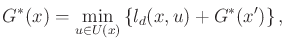(15.9)

in which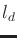is a discrete-time approximation to the cost that accumulates over stageand is given as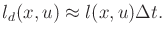(15.10)

It is assumed that asapproaches zero, the total discretized cost converges to the integrated cost of the continuous-time formulation.

Using the linear part of a Taylor series expansion about, the term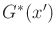can be approximated as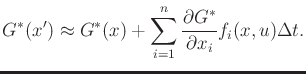(15.11)

This approximatesby its tangent plane at. Substitution of (15.11) and (15.10) into (15.9) yields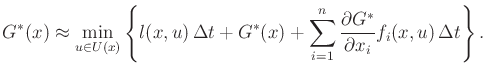(15.12)

Subtracting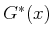from both sides of (15.12) yields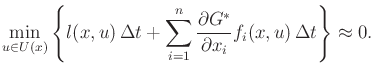(15.13)

Taking the limit asapproaches zero and then dividing byyields the HJB equation: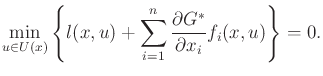(15.14)

Compare the HJB equation to (15.6) for the discrete-time case. Both indicate how the cost changes when moving in the best direction. Substitution of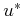for the optimal action into (15.14) yields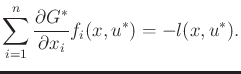(15.15)

This is just the continuous-time version of (15.8). In the current setting, the left side indicates the derivative of the cost-to-go function along the direction obtained by applying the optimal action from.

The HJB equation, together with a boundary condition that specifies the final-stage cost, sufficiently characterizes the optimal solution to the planning problem. Since it is expressed over the whole state space, solutions to the HJB equation yield optimal feedback plans. Unfortunately, the HJB equation cannot be solved analytically in most settings. Therefore, numerical techniques, such as the value iteration method of Section 14.5, must be employed. There is, however, an important class of problems that can be directly solved using the HJB equation; see Section 15.2.2.

Steven M LaValle 2020-08-14